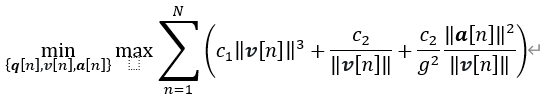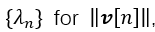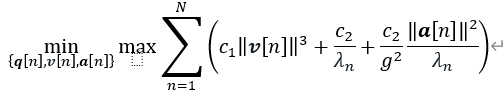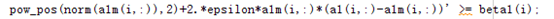# Status: Infeasible Optimal value (cvx_optval): +Inf

v[n] 、a[n] are Nx2,
lambda(N) refers to slack variable λ_n
beta(N) refers to slack variable β_n
1、My initial model is this:At first, I just introducing slack variables, and let the first-order Taylor of ‖v[n]‖ equals or greater thanso the function becomes likehere is my expression of target function2、But as for the third expression in the objective function‖a[n]‖^2/‖v[n]‖ ,even after I used quad_over_lin(pow_pos(norm(a(i,:)) ,2),lambda(i)),
It will appear “Cannot perform the operation {convex}.*{convex}” I
I think maybe my error is the same as this link Cannot perform the operation: {convex} .* {convex}, but it didn’t work .
3、so I introduced slack variables {β_n} to reformulate ‖a[n]‖^2, and let the first-order Taylor of ‖a[n]‖^2 equals or greater than β_n.
so the function becomes likeit still didn’t work.What’s more, if i just run the iteration for once,i can get some wrong numbers,error will appear if there are more times of iteration.Oh no…
here I post the work space, maybe can help you understand the structure

Please help me and let me know where the problem is!!! I will be very appreciated for you help

In the future, please copy and paste code using Preformatted text icon, rather than posting an image of code.

You should have `quad_iver_lin(...)`, not `inv_pos(...)*quad_over_lin`, beccause the `inv_pos` term is already included in `quad_over_lin`

quad_over_lin Sum of squares over linear.
Z=quad_over_lin(X,Y), where X is a vector and Y is a scalar, is equal to
SUM(ABS(X).^2)./Y if Y is positive, and +Inf otherwise. Y must be real.

``````If X is a matrix, quad_over_lin(X,Y) is a row vector containing the values
of quad_over_lin applied to each column. If X is an N-D array, the operation
is applied to the first non-singleton dimension of X.

quad_over_lin(X,Y,DIM) takes the sum along the dimension DIM of X.
A special value of DIM == 0 is accepted here, which is automatically
replaced with DIM == NDIMS(X) + 1. This has the effect of eliminating
the sum; thus quad_over_lin( X, Y, NDIMS(X) + 1 ) = ABS( X ).^2 ./ Y.

In all cases, Y must be compatible in the same sense as ./ with the squared
sum; that is, Y must be a scalar or the same size as SUM(ABS(X).^2,DIM).

Disciplined convex programming information:
quad_over_lin is convex, nonmontonic in X, and nonincreasing in Y.
Thus when used with CVX expressions, X must be convex (or affine)
and Y must be concave (or affine).``````

sorry,for some reason i can’t provide the whole code.I didn’t use inv_pos*quad_over_lin.I used them separately for two items

The invalid error is because your previous problem was infeasible, so the variable values were set to `nan`, which you apparently used as input to another (iteration) CVX problem, which considers `nan` input data in CVX expressions to be invalid.